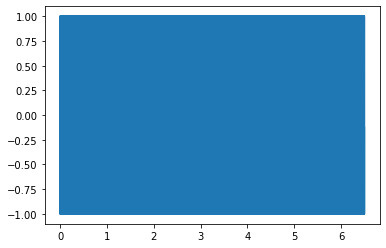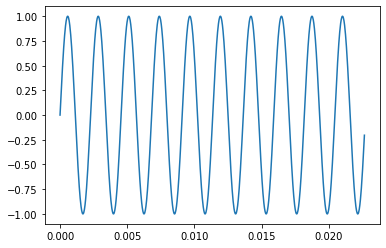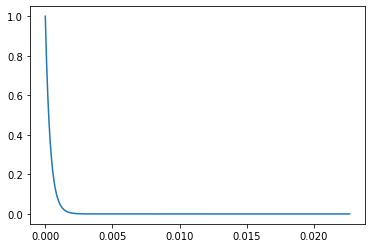:

from IPython.display import Audio

:

import numpy
import matplotlib.pyplot as plt
%matplotlib inline


# 39. What does a sinusoid sound like?¶

We all have advanced frequency detectors in our ears. This notebook will show how listening to signals gives you a whole different way to understand the frequency domain.

We start by constructing a musically relevant note as a pure sine wave

:

fs = 44100  # sampling frequency, Hz This is the rate at which CDs are sampled.
T = 0.5  # note length, seconds
A = 440  # Note frequency, Hz
twopi = 2*numpy.pi

t = numpy.linspace(0, T, int(T*fs), endpoint=False) # time variable
dt = t  # Sampling time
def note(frequency):
return numpy.sin(twopi*frequency*t)                # pure sine wave at 440 Hz

Audio(note(A), rate=fs)

:


Now we construct the Chromatic 12 note scale:

Chromatic scale

:

scalet = numpy.linspace(0, T*13, int(T*fs*13), endpoint=False)
scale = numpy.concatenate([note(A*2**(i/12.)) for i in range(13)])

:

Audio(scale, rate=fs)

:


Of course, this is quite a large number of points, you can’t really see what’s going on

:

plt.plot(scalet, scale)

:

[<matplotlib.lines.Line2D at 0x124602b70>]You can see the sinusoids better if you zoom in

:

Nzoom = 1000
plt.plot(scalet[:Nzoom], scale[:Nzoom])

:

[<matplotlib.lines.Line2D at 0x11b71b208>]Let’s listen to the effect of running this through a first order filter.

$G_f = \frac{1}{\tau s + 1}$

We’ll use the convolution, so we first obtain the impulse response of the filter

:

omega = 440*twopi  # (440 cycles/second)*(2 pi radians / cycle)
tau = 1/omega
first_order_impulse = numpy.exp(-t/tau)

:

plt.plot(t[:Nzoom], first_order_impulse[:Nzoom])

:

[<matplotlib.lines.Line2D at 0x11cc9cef0>]Then we calculate the output signal via convolution. Recall that when we wrote $$y(s) = G_f(s)u(s)$$, this was equivalent to calculating $$y(t) = g_f(t)*u(t)$$ where the $$*$$ represents convolution. In this case the filtered signal is $$y(t)$$ and the original scale is $$u(t)$$.

:

def filtersignal(signal):
return numpy.convolve(signal, first_order_impulse, 'same')/len(signal)*len(first_order_impulse)

:

filtered = filtersignal(scale)


As a side note: This is not how filtering is done in practice, you can see this process takes a while.

:

Audio(filtered, rate=fs)

: